May 7, 2021

## Q&A 43: Read first line of a file and move to a specific folder

Q: Read first line of a file and move to a specific folder. I have some files that sit on a server and I would like to move them off to archive. Each file has a code in the first line that tells me where the file came from. The files are regular text (.txt) files. How can I set up Limagito to move the file based on that code? If the code contains B01, move to a folder named Branch1. If the code contains B02, move to a folder named Branch2. The code will usually have more letters surrounding the B01 and B02, so I need the logic to pick up on B01 and B02 being in the code.
A: Yes this is possible. All you need a simple Pascal Script. We’ve added some screenshots to get you started.

As a start we added a new Moving Rule with WIN as Source. In the windows source folder we added some txt files. We’ll read the first line and check if certain codes exists (B01, B02, ..). Depending on the code found we’ll use a different subfolder of the Destination folder. If this subfolder doesn’t exist then we’ll create it.

1.Please open the Pascal Script option: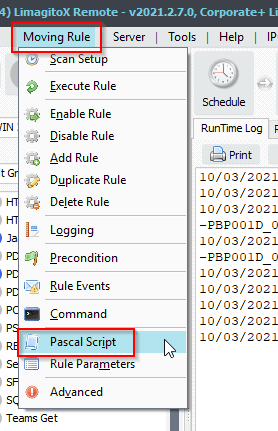2. Enable and add the following ‘On Destination’ Pascal Script:

```var
tmpLine: String;
tmpList: TStringList;
Begin
psExitCode:= 0;
psVSA := 'Unknown'
tmpList := TStringList.Create;
Try
try
// Check First Line
If tmpList.Count <> 0 Then
Begin
tmpLine := UpperCase(tmpList.Strings);
psLogWrite(1, '', 'First Line: ' + tmpLine);
// check
If Pos('B01', tmpLine) <> 0 Then psVSA := 'Alabama';
If Pos('B02', tmpLine) <> 0 Then psVSA := 'Alaska';
If Pos('B03', tmpLine) <> 0 Then psVSA := 'Arizona';
If Pos('B04', tmpLine) <> 0 Then psVSA := 'Arkansas';
If Pos('B05', tmpLine) <> 0 Then psVSA := 'California';
If Pos('B06', tmpLine) <> 0 Then psVSA := 'Colorado';
If Pos('B07', tmpLine) <> 0 Then psVSA := 'Connecticut';
If Pos('B08', tmpLine) <> 0 Then psVSA := 'Delaware';
If Pos('B09', tmpLine) <> 0 Then psVSA := 'Florida';
If Pos('B10', tmpLine) <> 0 Then psVSA := 'Georgia';
// Set ExitCode
psLogWrite(1, '', 'Subfolder which will be used ' + psVSA);
psExitCode := 1;
End
Else
psLogWrite(1, '', 'Error no lines in ' + psFilePath + psFileName);
Except
End;
Finally
tmpList.Free;
End;
End.```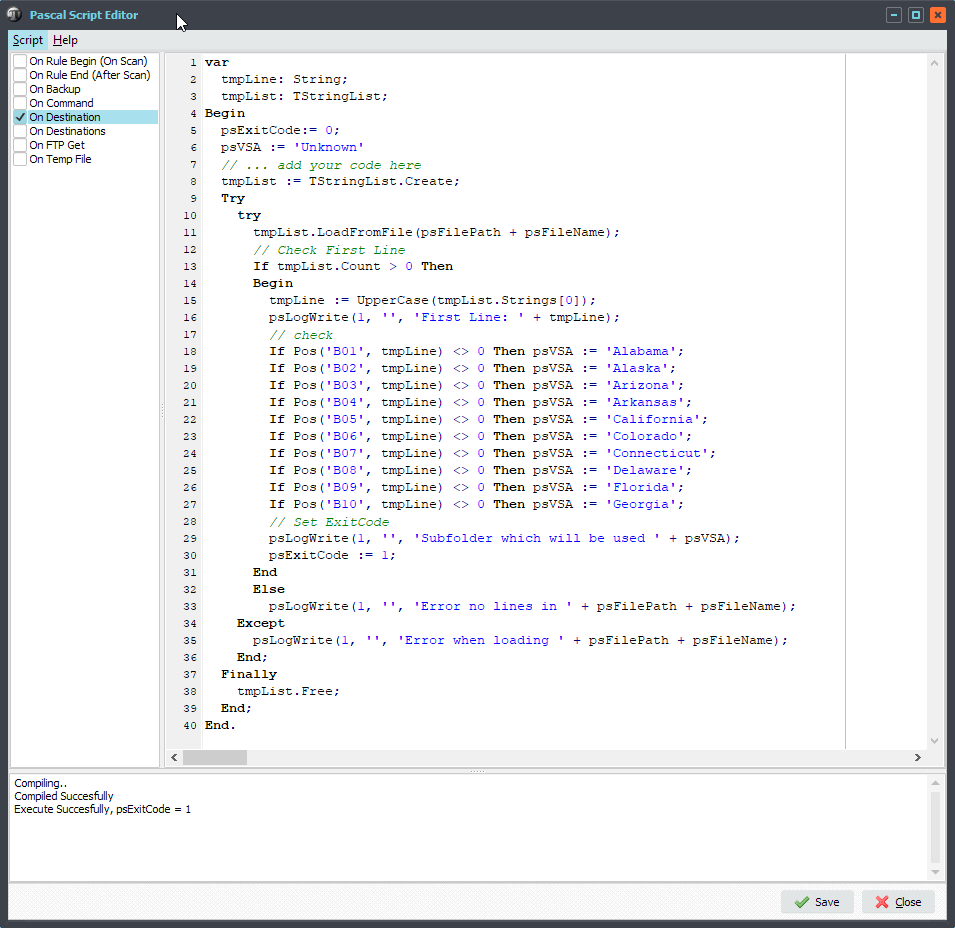3. Destination Setup, Create Subdir, option:  %VSA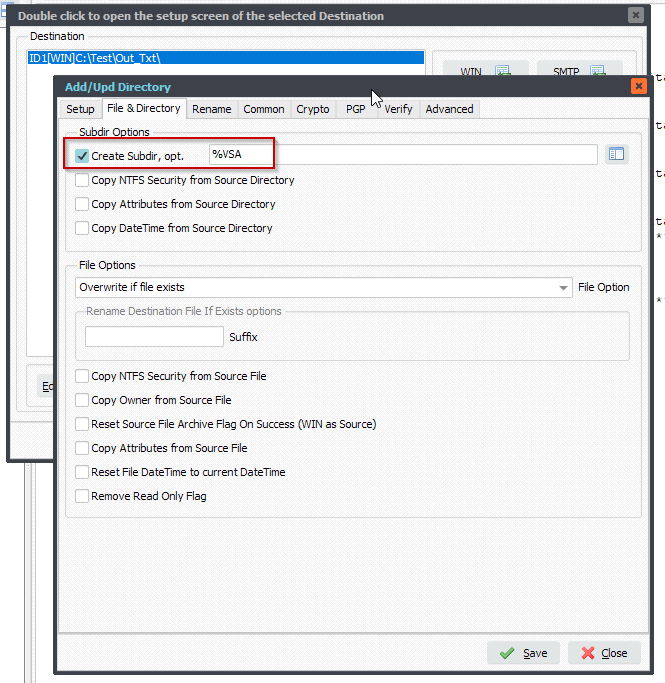4. RunTime Log Result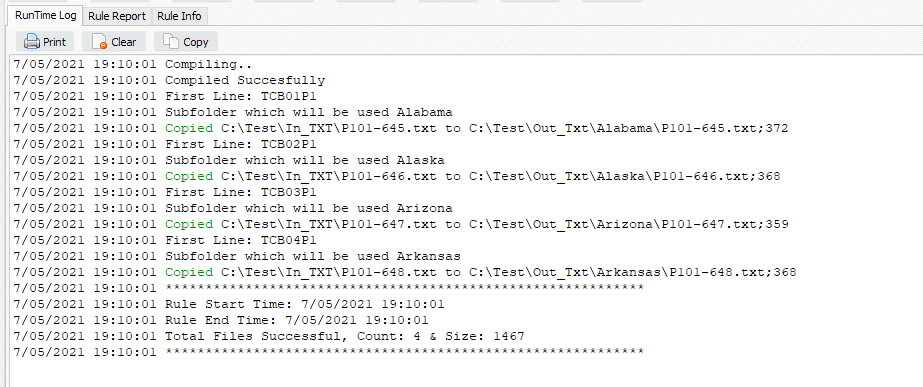5. Destination SubFolder Structure, if subfolder doesn’t exist then it will be create by our File Mover: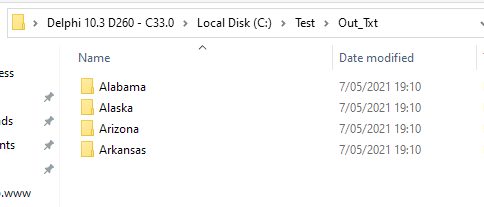#FileTransfer

If you need any help with this ‘Read first line of a file’ request, please let us know.

Happy Easter,

Limagito Team

By Limagito Share: